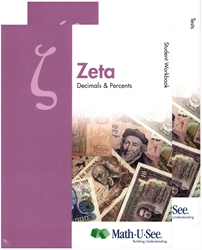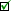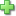# Math-U-See Zeta - Student Pack

## Decimals & Percents

Publisher: Math-U-See
2012 Edition, ©2012, Publisher Catalog #1061
Consumable Workbook
Price: \$48.00
Used Price: \$34.00 (1 in stock)Condition Policy

See series description for full review.

Zeta extends the student’s concept of place value to the right of the decimal point. Students learn to complete core operations with decimals. The connection between fractions and decimals is presented.

The Zeta Student Kit contains the Student Workbook with lesson-by-lesson worksheets, systematic review pages, and Application & Enrichment pages as well as Zeta Tests.

Scope and Sequence:

• Lesson 01: Exponents; Word Problem Tips
• Lesson 02: Place Value with Expanded and Exponential Notation
• Lesson 03: Decimal Numbers with Expanded Notation
• Lesson 04: Add Decimal Numbers
• Lesson 05: Subtract Decimal Numbers
• Lesson 06: Metric System Origin—Meter, Liter, Gram and Greek Prefixes; Multi-Step Word Problems
• Lesson 07: Metric System—Latin Prefixes
• Lesson 08: Metric System Conversion—Part 1
• Lesson 09: Multiply by 1/10 or 0.1
• Lesson 10: Multiply Decimals by 1/100 or 0.01
• Lesson 11: Finding a Percentage
• Lesson 12: Finding a Percentage > 100%; Word Problems
• Lesson 13: Reading Percentages in a Pie Graph
• Lesson 14: Multiply All Decimals
• Lesson 15: Metric System Conversions—Part 2
• Lesson 16: Computing Area and Circumference of a Circle
• Lesson 17: Dividing a Decimal by a Whole Number
• Lesson 18: Dividing a Whole Number by a Decimal; Word Problems
• Lesson 19: Solving for an Unknown
• Lesson 20: Dividing a Decimal by a Decimal
• Lesson 21: Decimal Remainders
• Lesson 22: More Solving for an Unknown
• Lesson 23: Convert Any Fraction to a Decimal and a Percentage
• Lesson 24: Decimals as Rational Numbers; Word Problems
• Lesson 25: Mean, Median, and Mode
• Lesson 26: Probability
• Lesson 27: Points, Lines, Rays, and Line Segments
• Lesson 28: Planes and Symbols
• Lesson 29: Angles
• Lesson 30: Types of Angles
Did you find this review helpful?
Series DescriptionClick here to write a review